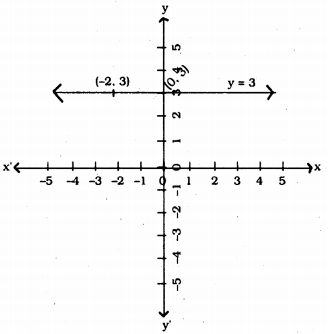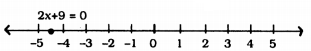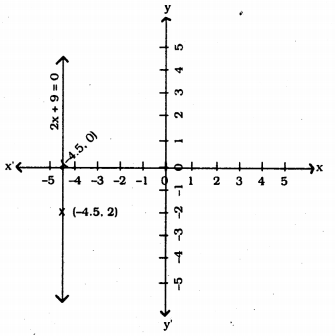# KSEEB Solutions for Class 9 Maths Chapter 10 Linear Equations in Two Variables Ex 10.4

KSEEB Solutions for Class 9 Maths Chapter 10 Linear Equations in Two Variables Ex 10.4 are part of KSEEB Solutions for Class 9 Maths. Here we have given Karnataka Board Class 9 Maths Chapter 10 Linear Equations in Two Variables Exercise 10.4.

## Karnataka Board Class 9 Maths Chapter 10 Linear Equations in Two Variables Ex 10.4

Question 1.
Give the geometric representations of y = 3 as an equation.
(i) in one variable
(ii) in two variables.
Solution:
x + 3 = 0
i) In one variable, y = 3.ii) In two variables, y = 3 straightline passes through (0, 3) parallel to x-axis. For any value of x, value of y is 3.Question 2.
Give the geometric representations of 2x + 9 = 0 as an equation.
(i) in one variable
(ii) in two variables.
Solution:
2x + 9 = 0, this equation in
i) One variable 2x + 9 =0
2x = -9
x = $$-\frac{9}{2}$$ = -4.5ii) 2x + 9 = 0 in two variables, 2x + 9 = 0. This equation passes through (-4.5, 0) and this is parallel ot y-axis. Coordinate of x = – 4.5We hope the KSEEB Solutions for Class 9 Maths Chapter 10 Linear Equations in Two Variables Ex 10.4 help you. If you have any query regarding Karnataka Board Class 9 Maths Chapter 10 Linear Equations in Two Variables Exercise 10.4, drop a comment below and we will get back to you at the earliest.

error: Content is protected !!